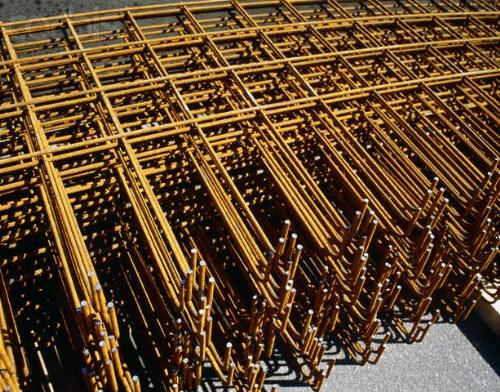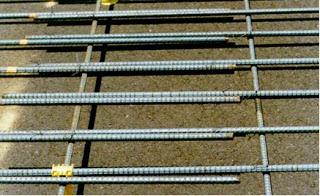How to calculate the over-Lapping length of reinforcement steel bars

# How to calculate the over-Lapping length of reinforcement steel barsNormally, steel bars have a 6m length. If length of a bar is not enough to keep the reinforcement, we have to lap  two steel bars. Normally, lapping position is at where near the minimum shear force is acting. Normally lap length is 50D meaning 50 times the bar diameter if both bars are of same diameter. When lapping two bars of different diameters, the lap length is considered as 50 times the smaller diameterSteel Lapping Formula Lap length = 50 X Diameter
How to calculate the over-lapping length of steel bars in slab,column and beam

Continue Reading about the "How to calculate the over-Lapping length of reinforcement steel bars" on the next page below